Hi folks! This is my first video for 2019 and I would like to wish everyone a great and fruitful year ahead!

In this video we want to discuss how to determine the number of monosubstituted products when an alkane undergoes free radical substitution.

We also want to estimate the expected ratio of these products.

We will be using 2 alkanes for examples: butane and 2-methylbutane.

Let's take a look at the first example butane:

The first thing is to determine the number of products when butane undergoes monosubstitution.

What we need to consider is this:

How many different types of carbon are there in this alkane?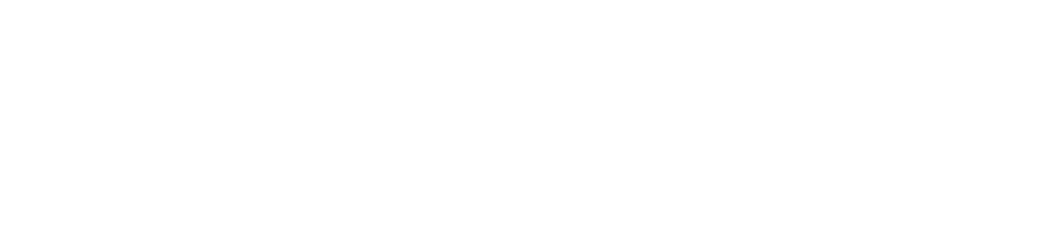From the structure of butane we can find 2 different types of carbon.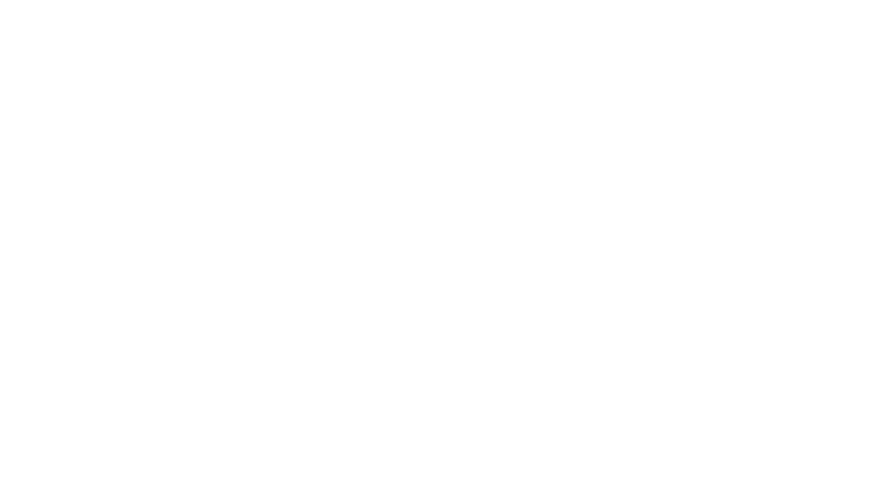So therefore we would expect 2 different monochloroalkane products: 1-chlorobutane and 2-chlorobutane.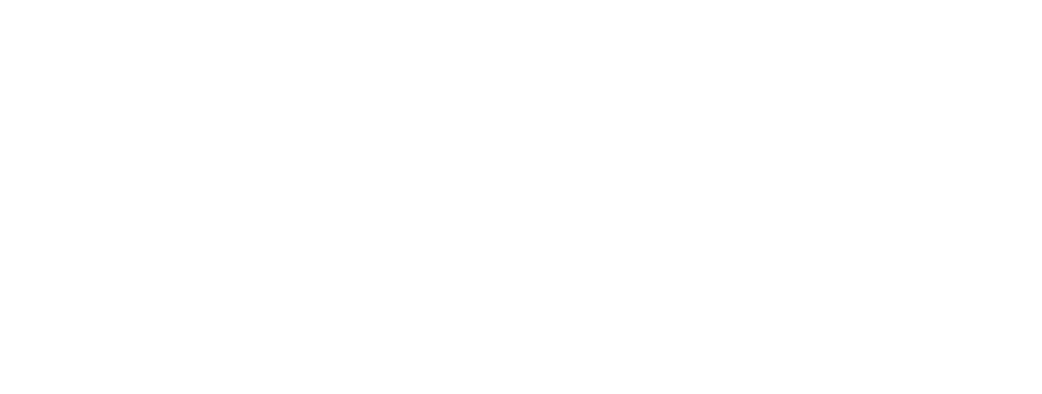Next we want to determine the ratio of these products.

Assuming the likelihood of substitution at each C-H bond is the same, we can then use probability to predict the ratio.

So what we focus on next is this:

How many C-H bonds are there for each type of carbon?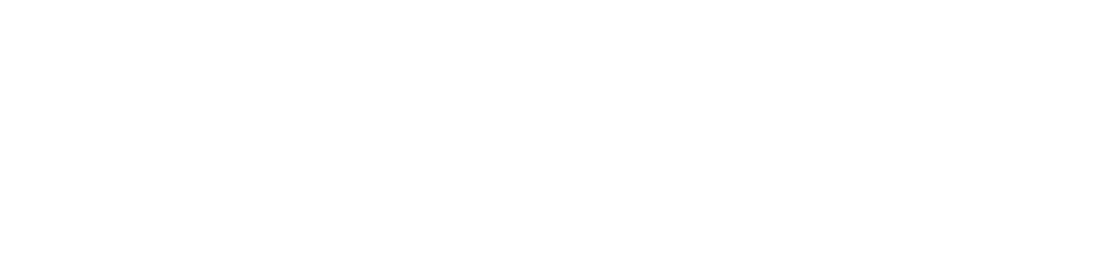By looking at the structure of butane, we can determine that substituting 6 C-H (blue) for Carbon-1 will form 1-chlorobutane and substituting 4 C-H (yellow) for Carbon-2 will form 2-chlorobutane.

So the ratio between 1-chlorobutane and 2-chlorobutane will be 6:4 or 3:2.

Let's consider the second example 2-methylbutane.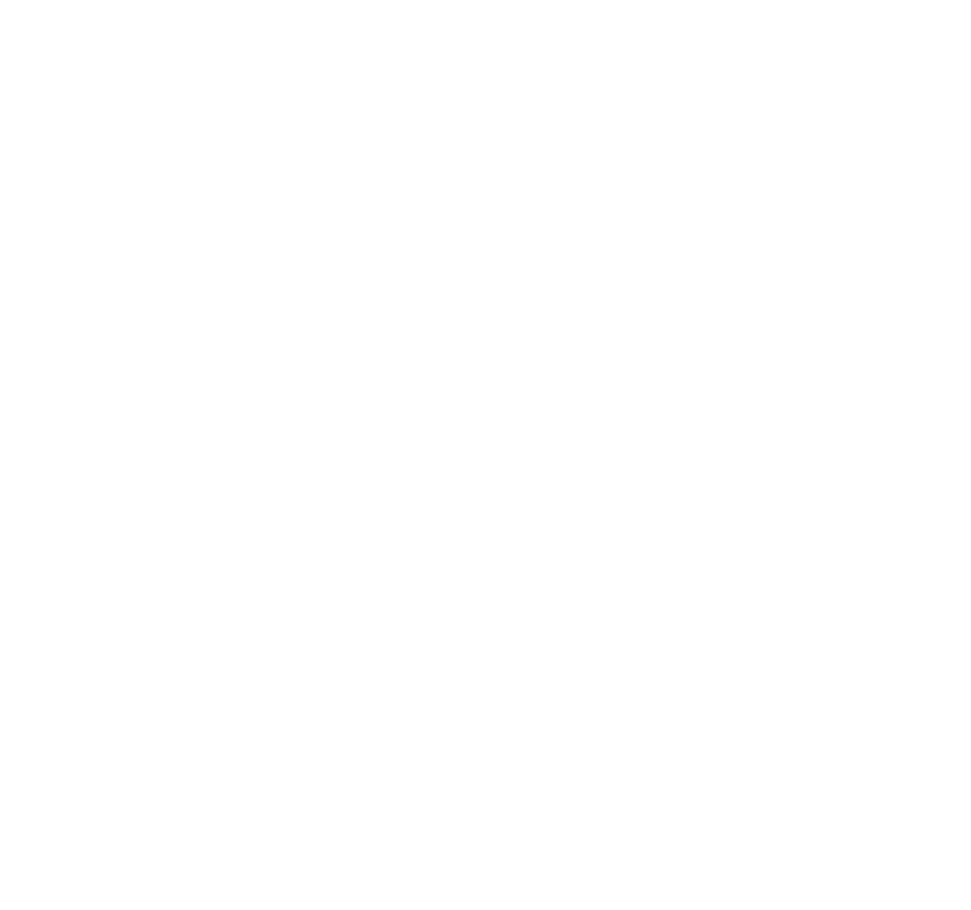There are 4 different types of carbon so there will be 4 monosubstituted products.

The number of C-H bonds for each type of carbon are listed below:

Carbon-1 has 6 C-H bonds (yellow)
Carbon-2 has 1 C-H bond (pink)
Carbon-3 has 2 C-H bonds (blue)
Carbon-4 has 3 C-H bonds (green)

So therefore the ratio of monosubstituted products 1, 2, 3 and 4 will be 6:1:2:3.

For the detailed step-by-step discussion on how to determine the number of free radical substitution products and their ratio, check out this video now!

Topic: Alkanes, Organic Chemistry, A Level Chemistry, Singapore

Back to other previous Organic Chemistry Video Lessons.